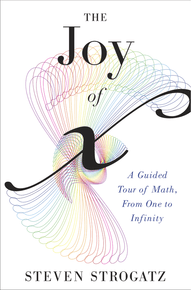I realise that this is not strictly a financial-literacy/economic-understanding type topic – but this book on math by Steven Strogatz was mind-blowing. For me, at least.

And actually, after consideration, I think it’s entirely relevant to the way one sees the finance world. It’s trite to put it this way: but mathematics is not just the functional tool for magnitude. It is art and philosophy and the language of God. Numbers are infinite, and irrational, and stretch wordlessly into the Deep Dark where pairs of prime numbers encounter each other in lengths of digits untouched by even the grandest of data-mining computer agglomerations. And it will ever be thus, for the number line remained and remains and will remain eternally unplumbed…

As I’ve mentioned before (in this post: Have you done the math? #SavingsAreImportant), we underestimate the role that our math bias (or, rather, anti-math bias) can have on our decision-making process.

We tend to underestimate the effect of compounding and overestimate the probability of good things happening. In the world of finance, great hedge funds have risen and fallen on the back of bad math (especially when it comes to option pricing models and that pesky fat tail on the Normal Distribution).

But I found that this book answered the questions I was asked constantly as a tutor: the “What’s the point of learning geometry?” and “When will I ever/When have I ever used the Sine curve?” variety.

It’s probably worth reading for that purpose alone.

Here is my favourite part…

The Unsolvable Sum

Take this series:

S = 1 – 1 + 1 – 1 + 1 – 1 + 1 …

Here’s the question: “what is S equal to”?

Well, all of us know that saying 3 + 7 is the same thing as saying 7 + 3, and that both of those equal to 10. It doesn’t really matter where you start when you’re just adding and subtracting.

So let’s start by inserting some brackets around the above and seeing if it does make a difference:

S = 1 – 1 + 1 – 1 + 1 – 1 + 1 …

S = (1 – 1) + (1 – 1) + (1 – 1) …

S = 0 + 0 + 0 …

S = 0

It looks like the answer is zero.

But wait, let’s try moving those brackets around a bit…

S = 1 – 1 + 1 – 1 + 1 – 1 + 1 …

S = 1 + (- 1 + 1) + (- 1 + 1) + (- 1 + 1) …

S = 1 + 0 + 0 + 0 …

S = 1

Could it be that the answer to that question is both 0 and 1?

Fact: the above was presented as mathematical proof of Creation. That is, from nothing (0), something (1). Here’s the actual quote from mathematician-priest Guido Grandi:

By putting parentheses in to the expression 1 – 1 + 1 – 1 + … in different ways, I can, if I want, obtain 0 or 1. But then, the idea of creation ex nihilo is perfectly plausible.

But actually, there’s another answer that works:

S = 1 – 1 + 1 – 1 + 1 – 1 + 1 …

S = 1 – [1 + 1 – 1 + 1 – 1 …]

but that bracketed part is S…

S = 1 – S

2S = 1

S = ½

Today, mathematicians talk about convergence. And they would say that the solution to S shifts between 0 and 1 depending on where you choose to stop calculating the sequence. Therefore, they would argue that the sequence does not converge, and that the sum of the infinite series is meaningless.

It all sounds a bit too logical and, well, atheistic if you ask me (I wanted to use the term “nihilistic”, but realised that would tend too much toward a zero answer).

Either way, this book is a delight.

And here’s a link to the youtube trailor clip by the author*:
*Just don’t be put off by him in person – his writing is much more exciting.